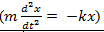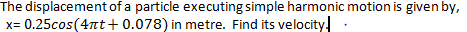Question FBQ1 :
 When the motion of particles of the medium is perpendicular to the direction in which the wave propagates, it is called a ____ wave.

Question FBQ2 : Two identical inductively coupled circuits, each having a natural frequency of 600 Hz, have coupling coefficient 0.44. Calculate the two normal mode frequencies.
Answer: 500 Hz and 802 Hz

Question FBQ3 : When a wave travels through a medium, the resistance to wave motion in a medium is called ____.

Question FBQ4 : In a_____ , the magnitude of restoring force is linearly proportional to the displacement

Question FBQ5 : What is the phase angle of this equation $x\left(t\right)=Acos\left({w}_{0}t+\phi \right)?$

Question FBQ6 : The restoring force is always directed towards the______of an oscillating body.

Question FBQ7 : _____ is a type of periodic motion where the restoring force is proportional to the displacement.

Question FBQ8 : In the case of simple harmonic motion (SHM), if the particle is at the mean position, then the particle is in____.

Question FBQ9 : The quantity k/m of the differential equation of a spring-mass system () has a dimension of ___________.

Question FBQ10 : The quantity k/m of the differential equation of a spring-mass systemhas a dimension of ___________.
The k/m in the above equation is replaced by angular frequency of the oscillatory motion, because

Question FBQ11 : When a system is said to be heavily damped, the motion of the system is said to be ______

Question FBQ12 : When b<wo, we refer to it as a case of ____ damping.

Question FBQ13 : $x\left(t\right)=mcos\left({w}_{0}t+\phi \right)$, the amplitude of this equation is _________.

Question FBQ14 : Amplitude is defined as _______.
Answer: Maximum displacement of an oscillating body

Question FBQ15 : Calculate the characteristic impedance offered by a thin wire of steel stretched by a force of 80 N weighing 2g per metre.

Question FBQ16 : Calculate the characteristic impedance offered by a thin wire of steel stretched by a force of 80 N weighing 2g per metre.

Question FBQ17 : What sound does our vocal cord create inside the throat when we talk?

Question FBQ18 : When a progressive wave reaches the boundary of a finite medium or an interface between two media, waves undergo_______________________________or/and _____.

Question FBQ19 : ______ is the minimum displacement of wave.

Question FBQ20 : The locus of points in the same phase at a particular time is called ___.

Question FBQ21 : Waves set up by a single, isolated disturbance are called ____

Question FBQ22 : The simplest type of a periodic wave is a ____wave.

Question FBQ23 : _____ are waves that occur at the boundary

Question FBQ24 : The displacement of a particle executing simple harmonic motion is given by, x= $0.25cos\left(4\pi t+0.078\right)$ in metre. The amplitude is_______.

Question FBQ25 :Question FBQ26 : The ____ waves govern the working of a radar for detection of aircrafts.

Question FBQ27 : When a wave moves from a lighter to a denser medium, its velocity ____

Question FBQ28 : The _____ conditions are the conditions which must be satisfied at the interface where the two media meet

Question FBQ29 : When Z2>Z1, the second string (medium) is denser, R12 is still ___, implying a phase change of $\pi$ on reflection.

Question FBQ30 : When resistance to motion is very strong, the system is said to be ___ damped.

Question FBQ31 : If the source of a wave is so far from away from an aperture that the wavefront generating the diffraction pattern is regarded as plane wavefront, we have _____ diffraction

Question FBQ32 : The waves produced by a motor boat sailing in water are___.

Question FBQ33 : ________is the superposition of many waves of same amplitude and frequency, but differing slightly in phase.

Question FBQ34 : The statement that every point on an advancing wave front is a source of secondary wavelet is _____ principle.

Question FBQ35 : The intensity of a wave is the measure of its ___across a unit area perpendicular to the direction of motion.

Question MCQ1 : Which of the following is/are an example of a mechanical wave (I) Sound wave (II) Water waves (III) Light waves

Question MCQ2 : Which of the following is not a property of a longitudinal wave?

Question MCQ3 : The frequency of wave is 0.002 Hz. Its time period is ________.

Question MCQ4 : A pendulum suspended from the roof of a train has a period T (When the train is at rest). When the train is accelerating with a uniform acceleration ‘a’, the time period of the pendulum will ________.

Question MCQ5 : In simple harmonic motion, velocity at equilibrium position is ________.

Question MCQ6 : Over-damping results to ________.

Question MCQ7 : In simple harmonic motion (SHM), the particle is:

Question MCQ8 : A damped system is characterised by all of the following except ________.

Question MCQ9 : The total energy of a particle executing SHM is proportional to ________.
Answer: square of amplitude of motion

Question MCQ10 : Which of the following options is incorrect of damping motion?

Question MCQ11 : Which of the following represent stokes law?
Answer: 6$\pi$ηrv

Question MCQ12 : In the equation Fd= -ɣv, the negative sign indicates that ______.
Answer: the damping force opposes motion

Question MCQ13 : A vibration of a pendulum in a viscous medium such as thick oil is an example of ________.

Question MCQ14 : For a simple harmonic oscillator, the number of vibrations executed per second is called _______________

Question MCQ15 : The intensity of a wave is the measure of its ________.
Answer: power across a unit area perpendicular to the direction of motion

Question MCQ16 : A student tunes a guitar by comparing the sound of the string with that of a standard tuning fork. He notices a beat frequency of 5 Hz when both sounds are superposed. He tightens the guitar string and finds the beat frequency rises to 8 Hz. What should he do to match the frequency of the string to that of the tuning fork?
Answer: He must loosen the guitar string

Question MCQ17 : A note of frequency 1200 vibrations/s has an intensity of 2.0µW/m2. What is the amplitude of the air vibrations caused by this sound?

Question MCQ18 : When the motion of particles of the medium is along the direction in which wave propagates, it is called a ____.

Question MCQ19 : Oscillations become damped due to ________.

Question MCQ20 : The time period of a pendulum on Earth is 1.0 s. What would be the period of a pendulum of the same length on a planet with half the density but twice the radius of Earth?

Question MCQ21 : Two sound waves have intensities 0.4 and 10W/m2, respectively. How many decibels is one louder than the other?

Question MCQ22 : A simple pendulum has a period of 2 s and an amplitude of 50. After 20 complete oscillations, its amplitude is reduced to 40. Find the damping constant and the time constant.

Question MCQ23 : The quality factor of a sonometer wire is 4,000. The wire vibrates at a frequency of 300 Hz. Find the time in which the amplitude decreases to half of its original value.

Question MCQ24 : What is the ratio of the wavelength to the period of a wave?

Question MCQ25 : A box of mass 0.2 kg is attached to one end of a spring whose other end is fixed to a rigid support. When a mass of 0.8 kg is placed inside the box, the system performs 4 oscillations per second and the amplitude falls from 2 cm to 1 cm in 30 sec. Calculate the relaxation time.

Question MCQ26 : A box of mass 0.2 kg is attached to one end of a spring whose other end is fixed to a rigid support. When a mass of 0.8 kg is placed inside the box, the system performs 4 oscillations per second and the amplitude falls from 2 cm to 1 cm in 30 sec. Calculate the quality factor.

Question MCQ27 : The quality factor of a tuning fork of frequency 512Hz is 610^4. Calculate the time in which its energy is reduced to e-1 of its energy in the absence of damping.

Question MCQ28 : The quality factor of a tuning fork of frequency 512Hz is 610^4. How many oscillations will the tuning fork make in this time?

Question MCQ29 : As amplitude of resonant vibrations decreases, degree of damping ______.

Question MCQ30 : An electric bell has a frequency 100Hz. If its time constant is 2s, determine the Q factor for the bell.

Question MCQ31 : The dot or scalar product of a force and a displacement vectors defines______.